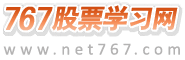767股票学习网 > 股票书籍 > 怎样编写大智慧指标公式 > 正文

# 创历史新高

（12）、创历史新高　　　hhv(close,0)=close; 注：hhv(x,n),求n周期内x最高值,n=0则从第一个有效值开始。（13）、创历史新低　　　llv(close,0)=close; 注：llv(x,n),求n周期内x最小值,n=0则从第一个有效值开始。（14）、单日放量　　　a:=ref(ma(vol,5),1); vol/a>n and vol/capital*100>m; 注：n为指标参数,代表今日成交量和前一日的5日均量的比值,可在参数设定范围内设定参数范围。（15）、阶段缩量　　　sum(vol,n1)/capital<=n2; 注：n1为要统计的阶段时间,n2为缩量比例,可在参数设定范围内设定参数范围。（16）、阶段放量　　　sum(vol,n1)/capital>=n2; 注：n1为要统计的阶段时间,n2为y放量比例,可在参数设定范围内设定参数范围。（17）、持续放量　　　a:=vol>ref(vol,1); count(a,n)=n; 注：其中条件a的含义为成交量较上一交易日放大,n为要统计的阶段时间,可在参数设定范围内设定参数范围。（18）、持续缩量　　　a:=vol< ref(vol,1); count(a,n)=n; 注：其中条件a的含义为成交量较上一交易日缩小,n为要统计的阶段时间,可在参数设定范围内设定参数范围。（19）、间隔放量　　　a:=ma(vol,5); barscount(c)>=n and hhv(a,n)< n1*llv(a,n) and count(vol>n2*a,n)>n3; 注：a.barscount(x)第一个有效数据到当前的天数。Barscount(c)对于日线数据取得上市以来总交易日数。 b.完整含义：n日内五日均量的最大值小于最小值的n1倍,成交量大于等于五日均量的n2倍的情况发生的次数多于n3次。（20）、放量上攻 a:=(c-ref(c,1))/ref(c,1)>=(n/100); sum(vol,n1)/capital>=(n2/100) and count(vol>ref(vol,1),n3 )=n3 and count(a,n3)=n3; 完整含义：每日股价涨幅均大于n%,n1日内累计换手率大于n2%,并且成交量连续在n3日内放大。（21）、温和放量上攻　　　股价小幅上扬 a1:=close/ref(close,1); a2:=a1>1 and a1<1.03; 成交量小幅上扬　　　b1:=vol/ref(vol,1); b2:=b1>1 and b1<2; c1:=ma(vol,n)/capital<0.05; count(a2 and b2,n)/n>0.6 and c1; 完整公式为：　　　a1:=close/ref(close,1); a2:=a1>1 and a1<1.03; b1:=vol/ref(vol,1); b2:=b1>1 and b1<2; c1:=ma(vol,n)/capital<0.05; count(a2 and b2,n)/n>0.6 and c1; 注：公式分为股价小幅上扬和成交量小幅上扬两个部分,请在指标编写时注意其结构。（22）、突然放量　　　vol>ref(hhv(vol,n),1*m; 注：当前成交量高于n个周期中最大成交量的m倍。（23）、平台整理　　　count(close,0)>n and (hhv(close,n)-llv(close,n))/llv(close,n)<=(n1/100); 注：count(close,0)>n为平台整理n个周期的设定。(hhv(close,n)-llv(close,n))/llv(close,n)<=(n1/100)为平台整理幅度n1%的设定。

 上一篇文章： 走势特征选股公式的编制 下一篇文章： 突破长期盘整

 767股票学习网　|　手机版　|　微信　|　微博　|　联系站长